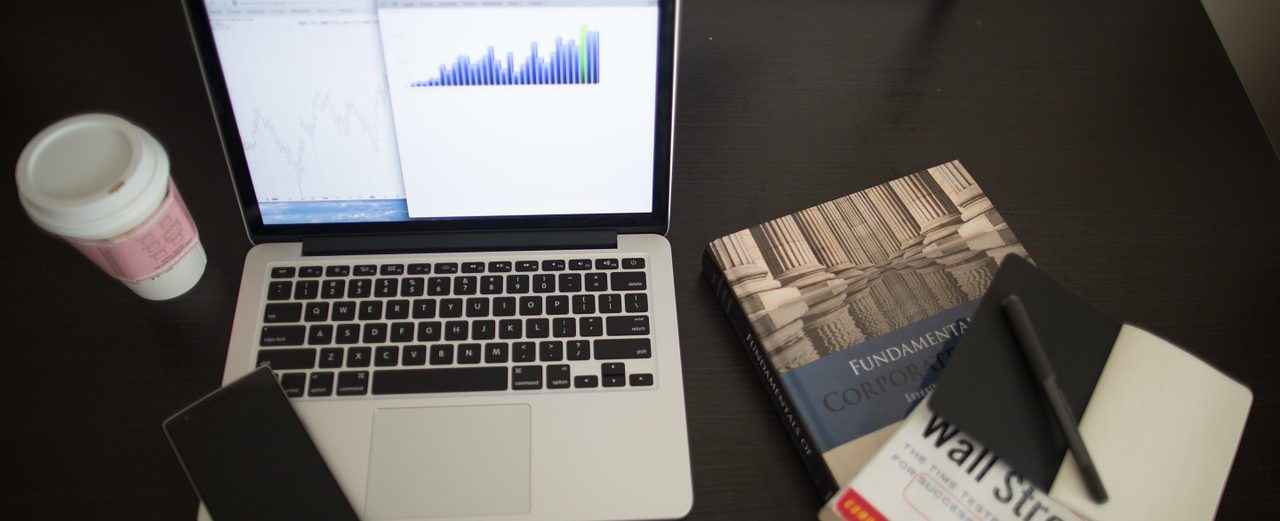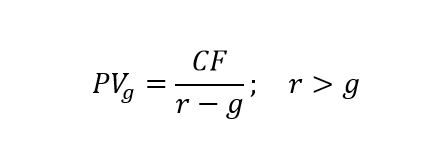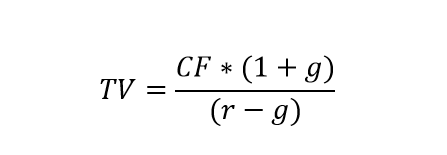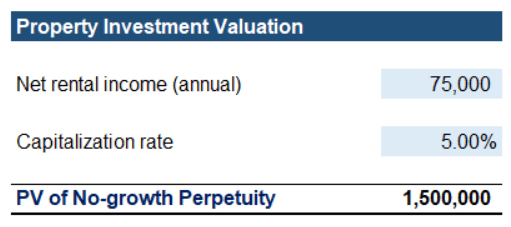## Introduction

The Perpetuity concept refers to the present value (PV) of equal periodic cash flows that investors will receive over an indefinite future period.

We need to calculate the present value of perpetual cash flows for a variety of reasons, some being:

• Companies have cash flow projections for a reasonable number of periods and no other way to value the indefinite future performance of the business;
• Some financial instruments like bonds and preferred dividends pay coupons forever, and investors need a way to evaluate such.

In business and finance, perpetuity is a regular payout of the same amount with no end.

## Perpetuity Concept

Perpetuity is a form of an ordinary annuity, with no end, a stream of cash payments that carries on forever. We also refer to it as a perpetual annuity. The method is one of the time value of money techniques employed in financial assets valuation.

The concept is closely related to terminal value and terminal growth rate in valuation modeling. It is fundamental to the Dividend Discount Model (DDM), used to evaluate the price of common shares.

It represents fixed payments on permanently invested amounts, meaning that the principal is irredeemable.

The value of a perpetuity is finite, and we can calculate it because payments far in the future start to have present values close to zero. And as the principal never gets repaid, we do not have the issue with calculating its current value.### No-growth Perpetuity

It seems void of logic, but infinite cash flows in the future can have a finite present value. As we discussed in our article on the Time Value of Money, marginal increases in payouts decrease over time. Eventually, the future value of a perpetual annuity payment is equal to the future value of an ordinary annuity, which matures at that point in the future. This means that each payment is only a fraction of the last.

To calculate perpetuity, we apply the following formula:We can also present the present value mathematically by the sum of all future cash flows for an infinite number of periods.Where:

• CF is the constant cash flow;
• n is the number of the period;
• r is the discount rate.

A simple mathematical test can lead to a simplified formula. Let’s assume we are looking at an annuity’s present value. The method to calculate an ordinary annuity is:We can look at part of the formula:Calculating (1 + r) to the power of n when n is infinite gets closer to infinity with each iteration. Therefore we can conclude that the multiplier closes to zero in infinity. We are then left with the following formula when we replace it:And from here, we arrive at the present value of no-growth perpetuity.### Constant-growth Perpetuity

Constant-Growth perpetuity also pays out forever, but payments increase over time at a constant growth rate.This version of perpetuity is a popular method for valuing traditional dividend-paying stocks by financial institutions and large industrial companies. It used to be very popular when the majority of businesses used dividends as a primary method to distribute profit to shareholders.

In a similar fashion to the calculation of ordinary perpetuity, we can arrive at the present value of constant-growth perpetuity, where the assumed growth is always lower than the discount factor.We mostly apply the concept in Terminal Value (TV) calculation, which is used in financial modeling and valuation. Perpetuity determines the cash flows in the final year of the business. In finance, a company is considered a ‘going concern,’ i.e., it goes on indefinitely. Therefore financial analysts use the concept of perpetuity to calculate the terminal value of the company.

The formula for terminal value is a bit more complicated:Where:

You can read more in our article on the Terminal Value of the Business.

## Uses in Real LifeThere are two real-life examples where the concept of perpetuity is mostly applied.

The first one is in the valuation of properties in the real estate sector. Applying perpetuity, we can assume that the value of a property is its cash in-flow (or net rental income), divided over the capitalization rate. We use the capitalization rate as a discount factor, as it shows the potential rate of return on the real estate investment.

There are two underlying assumptions in this scenario:

• Rents will increase with the same rate as inflation;
• Property may be sold soon, but the expectation is that the next investor will apply the same valuation approach.

The second real-life case is when we apply the constant growth Dividend Discount Model for the valuation of companies’ common stock.

The underlying assumption is that the market price per share is equal to the present value of all future dividends that will be received. This approach also considers future dividend payments to be perpetual.

If we have a discount rate of 12% and an expected dividend payout of 120 euro at the end of each period, the present value of the perpetual dividend payout will be 1,000 euro.

If we apply an expected constant growth rate of the dividend at 2%, we then get a present value of the perpetuity at the amount of 1,200 euro (120 euro / (12% – 2%)).

## Example

To better understand the concept, let us take a look at a simple example. We have the opportunity to invest 1.2 mil euro in real estate property. Our investment will bring us a perpetual 75 thousand euro annual net rental income. The capitalization rate for similar properties in the region is 5%. Remember, we ignore inflation and yearly growth as we believe them to cancel each other out. The calculation leaves us with a present value of 1.5 mil euro for the property. If we are basing our decision solely on perpetuity, we will choose to invest.Let also take a look at applying the Dividend Discount Model to estimate the value of a stock. We have the opportunity to invest 60 thousand euros in a business that will pay out a perpetual preferred dividend at the amount of 4,500 euros. The discount rate is 11.50% (our cost of capital), and we assume a constant growth rate of the dividend cash flows at 3%.

Applying the discussed calculations, we arrive at less than 55 thousand euros. It turns out this is not a viable investment opportunity, as we need to pay more than the present value of all future dividends.## Conclusion

The perpetuity concept reflects an infinite stream of equal cash flows received at regular intervals over time. It is applied mostly in the valuation of investments that have close to indefinite life spans, as real estate property and companies’ common stock. The method is fundamental to the Dividend Discount Model for valuation and the calculation of the Terminal Value of the Business.

If you liked this article, you could help by sharing it with colleagues and friends.

## And Get a FREE Benchmark Analysis Template## FCCA, FMVA

Hi! I am a finance professional with 10+ years of experience in audit, controlling, reporting, financial analysis and modeling. I am excited to delve deep into specifics of various industries, where I can identify the best solutions for clients I work with.

In my spare time, I am into skiing, hiking and running. I am also active on Instagram and YouTube, where I try different ways to express my creative side.